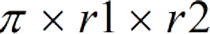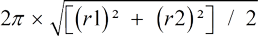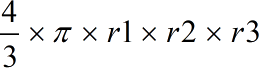Request a Tool

Area of an ellipse Calculator

This tools make it easy to calculate the area, perameter and volume of ellips.

Area
0

Formula• π = a constant value 3.1415
• r1 = Radius of the ellipse
• r2 = Radius of the ellipse

Defination / Uses

Ellipse is a plane curve formed by the intersection of a cone and a plane, resulting in a closed curve. An ellipse is a set of points in a plane where the distances between two fixed points add up to a constant.

An ellipse is a curved line that forms a closed loop and has a constant sum of distances between two points (foci) and every point on the line. An ellipse is usually defined as the bounded case of a conic section.

Perameter
0

Formula• π = a constant value 3.1415
• r1 = Radius of the ellipse
• r2 = Radius of the ellipse

Defination / Uses

Ellipse is a plane curve formed by the intersection of a cone and a plane, resulting in a closed curve. An ellipse is a set of points in a plane where the distances between two fixed points add up to a constant.

An ellipse is a curved line that forms a closed loop and has a constant sum of distances between two points (foci) and every point on the line. An ellipse is usually defined as the bounded case of a conic section.

Volume
0

Formula• π = a constant value 3.1415
• r1 = Radius of the ellipse
• r2 = Radius of the ellipse
• r3 = Radius of the ellipse

Defination / Uses

Ellipse is a plane curve formed by the intersection of a cone and a plane, resulting in a closed curve. An ellipse is a set of points in a plane where the distances between two fixed points add up to a constant.

An ellipse is a curved line that forms a closed loop and has a constant sum of distances between two points (foci) and every point on the line. An ellipse is usually defined as the bounded case of a conic section.

How to use ellipse calcultor?

There are only two basic steps.

• First select the option from dropdown. What you need to calculate.
• Then add the other values in their corresponding fields.

Thats it! You will get your answer in just a second. use upper given formula in case of manual calculation

Ellipse area calculator is a sophisticated online tool for calculating an ellipse's area. It estimates the ellipse area using only the user's major and minor radius. An ellipse is a closed curve on a plane that can be made by intersecting a plane with a circular cylinder or by projecting a circle onto a plane orthogonally. An ellipse is a specific instance of a circle. To calculate the area of an ellipse, we must first determine the length of the two semiaxes, or the length of the ellipse's two axes. We can use the formula to compute the area of an ellipse if we know the indicated numbers.